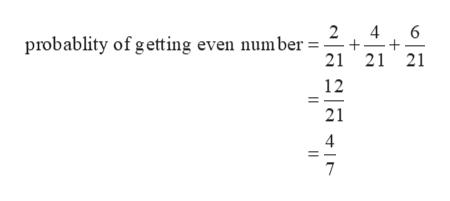# A die is loaded in such a way that the probability of each face turning up is proportional to the number of dots on that face. (For example, a six is three times as probable as a two.) What is the probability of getting an even number in one throw?

Question
240 views

A die is loaded in such a way that the probability of each face turning up is proportional to the number of dots on that face. (For example, a six is three times as probable as a two.) What is the probability of getting an even number in one throw?

check_circle

Step 1

The probability of each face turning up is proportional to the number of dots on that face so,

The probability of getting 1 is p

The probability of getting 2 is 2p

The probability of getting 3 is 3p

The probability of getting 4 is 4p

The probability of getting 5 is 5p

and The probability of getting 6 is 6p.

Since by the probability rule, sum of probabilities of the all the possible outcomes is equal to 1. So

Step 2

The probability of getting an even number in one throw = probability of getting 2 + probability...help_outlineImage Transcriptionclose4 6 2 probablity of getting even number 21 21 21 12 21 4 7 fullscreen

### Want to see the full answer?

See Solution

#### Want to see this answer and more?

Solutions are written by subject experts who are available 24/7. Questions are typically answered within 1 hour.*

See Solution
*Response times may vary by subject and question.
Tagged in

### Basic Probability